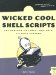9 An Arbitrary-Precision Floating-Point Calculator

#9 An Arbitrary-Precision Floating-Point Calculator

One of the most commonly used sequences in script writing is \$(()) , which lets you perform calculations using various rudimentary mathematical functions. This sequence can be quite useful, most commonly when incrementing counter variables , and it supports addition, subtraction, division, remainder, and multiplication, though not any sort of fractional or decimal value. Thus, the following command returns 0, not 0.5:

echo \$((1 / 2))

So when calculating values that need better precision, you've got a challenge on your hands. There just aren't many good calculator programs that work on the command line. Except, that is, for bc , an oddball program that few Unix people are taught. Billing itself as an arbitrary-precision calculator, the bc program harkens back to the very dawn of Unix, with its cryptic error messages, complete lack of prompts, and assumption that if you're using it, you already know what you're doing. But that's okay. We can cope.

The Code

#!/bin/sh # scriptbc - Wrapper for 'bc' that returns the result of a calculation. if [  = "-p" ] ; then   precision=   shift 2 else   precision=2           # default fi bc -q << EOF scale=\$precision \$* quit EOF exit 0

How It Works

This script demonstrates the useful here document capability in shell scripting. The << notation allows you to include material in the script that is treated as if it were taken directly from the input stream, which in this case allows an easy mechanism for handing commands to the bc program.

This is also our first script that demonstrates how command arguments can be utilized within a script to enhance the flexibility of a command. Here, if the script is invoked with a -p flag, it allows you to specify the desired scale. If no scale is specified, the program defaults to scale=2 .

When working with bc , it's critical to understand the difference between length and scale . As far as bc is concerned , length refers to the total number of decimal digits in the number, while scale is the total number of digits after the decimal point. Thus, 10.25 has a length of four and a scale of two, while 3.14159 has a length of six and a scale of five.

By default, bc has a variable value for length , but because it has a scale of zero, bc without any modifications works exactly as the \$(()) notation does. Fortunately, if you add a scale setting to bc , you find that there's lots of hidden power under the hood, as shown here:

\$  bc  bc 1.05 Copyright 1991, 1992, 1993, 1994, 1997, 1998 Free Software Foundation, Inc. This is free software with ABSOLUTELY NO WARRANTY. For details type `warranty'.  scale=10   (2002-1962)*365  14600  14600/7  2085.7142857142 quit

To allow access to the bc capabilities from the command line, a wrapper script has to silence the opening copyright information, if present, even though most bc implementations know that they should silence the header if their input isn't the terminal ( stdin ). The wrapper also sets the scale to a reasonable value, feeds in the actual expression to the bc program, and then exits with a quit command.

Running the Script

To run this script, feed a mathematical expression to the program as an argument.

The Results

\$  scriptbc 14600/7  2085.71 \$  scriptbc -p 10 14600/7  2085.7142857142Wicked Cool Shell Scripts
ISBN: 1593270127
EAN: 2147483647
Year: 2004
Pages: 150
Authors: Dave Taylor

Similar book on Amazon